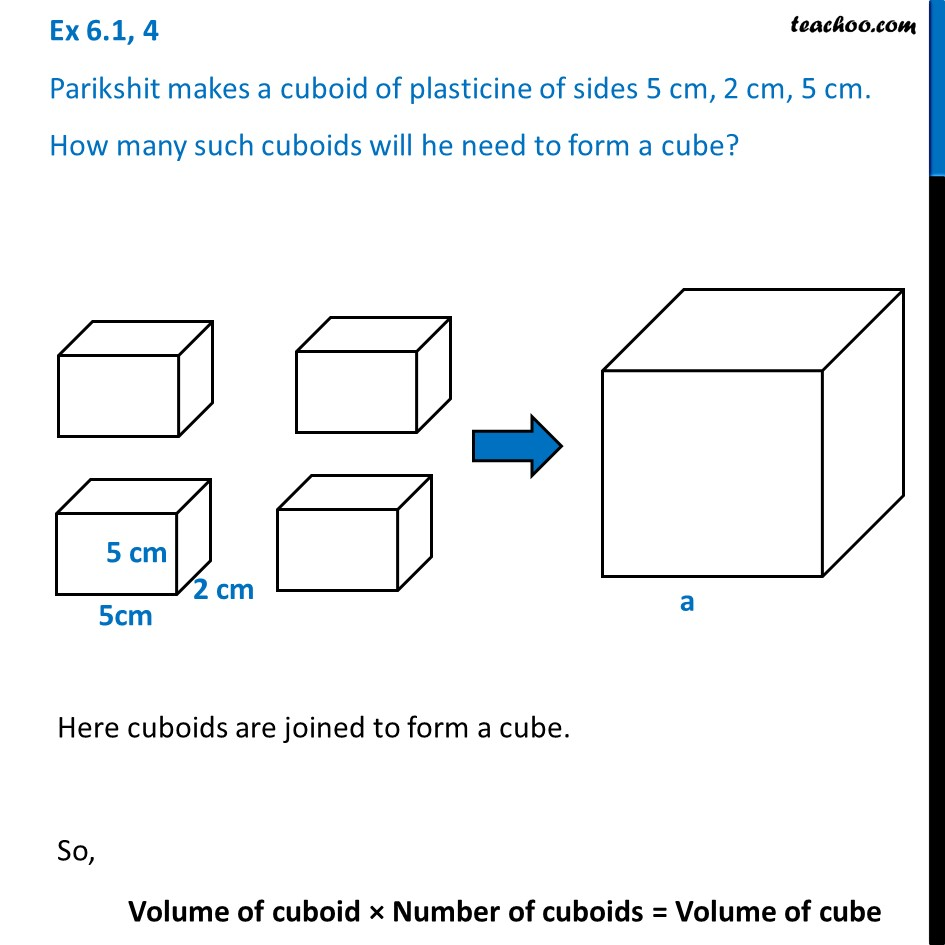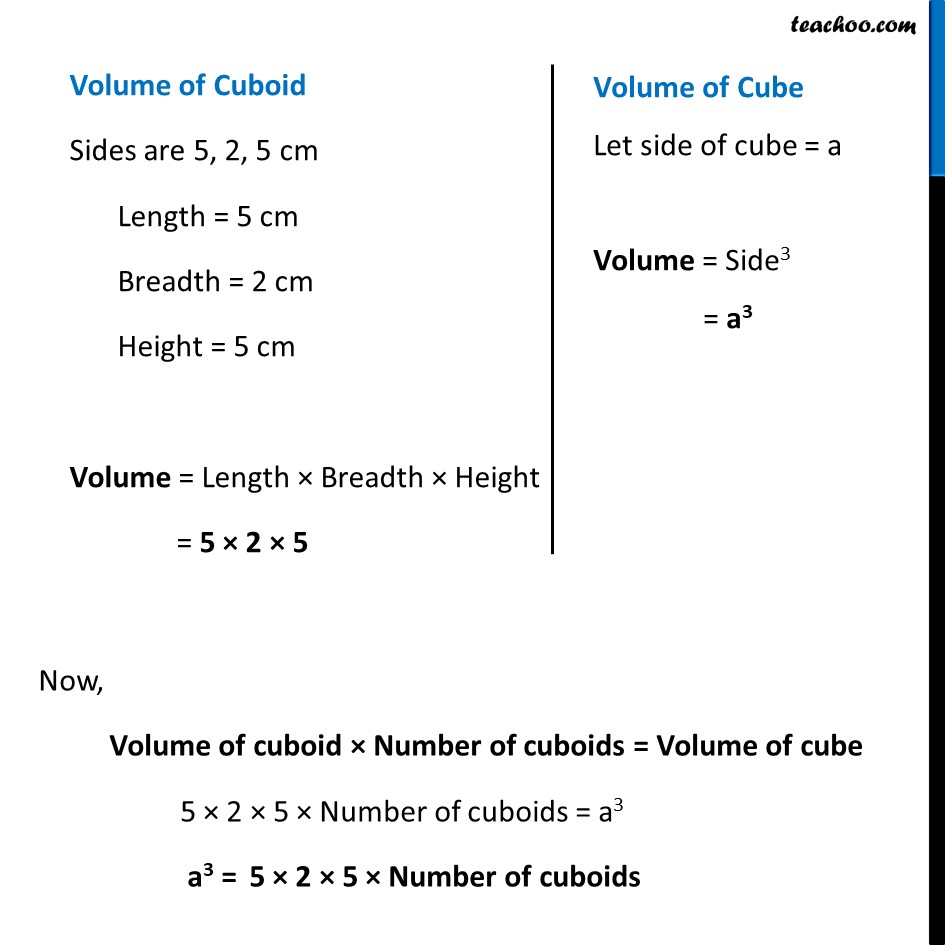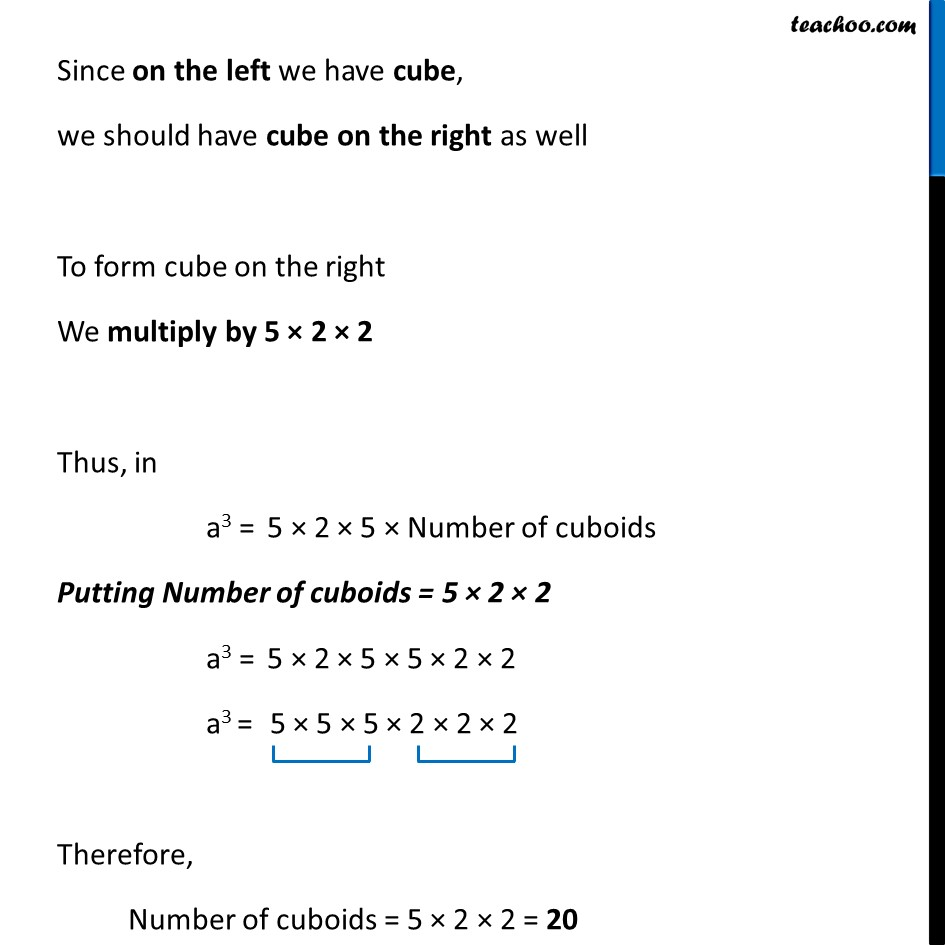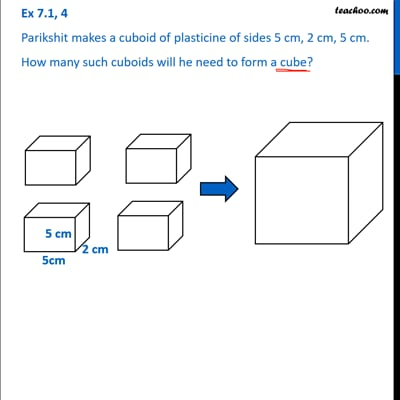Ex 6.1

Chapter 6 Class 8 Cubes and Cube Roots
Serial order wiseThis video is only available for Teachoo black users

Learn in your speed, with individual attention - Teachoo Maths 1-on-1 Class

### Transcript

Ex 6.1, 4 Parikshit makes a cuboid of plasticine of sides 5 cm, 2 cm, 5 cm. How many such cuboids will he need to form a cube? Here cuboids are joined to form a cube. So, Volume of cuboid × Number of cuboids = Volume of cube Volume of Cuboid Sides are 5, 2, 5 cm Length = 5 cm Breadth = 2 cm Height = 5 cm Volume = Length × Breadth × Height = 5 × 2 × 5 Volume of Cube Let side of cube = a Volume = Side3 = a3 Now, Volume of cuboid × Number of cuboids = Volume of cube 5 × 2 × 5 × Number of cuboids = a3 a3 = 5 × 2 × 5 × Number of cuboids Since on the left we have cube, we should have cube on the right as well To form cube on the right We multiply by 5 × 2 × 2 Thus, in a3 = 5 × 2 × 5 × Number of cuboids Putting Number of cuboids = 5 × 2 × 2 a3 = 5 × 2 × 5 × 5 × 2 × 2 a3 = 5 × 5 × 5 × 2 × 2 × 2 Therefore, Number of cuboids = 5 × 2 × 2 = 20